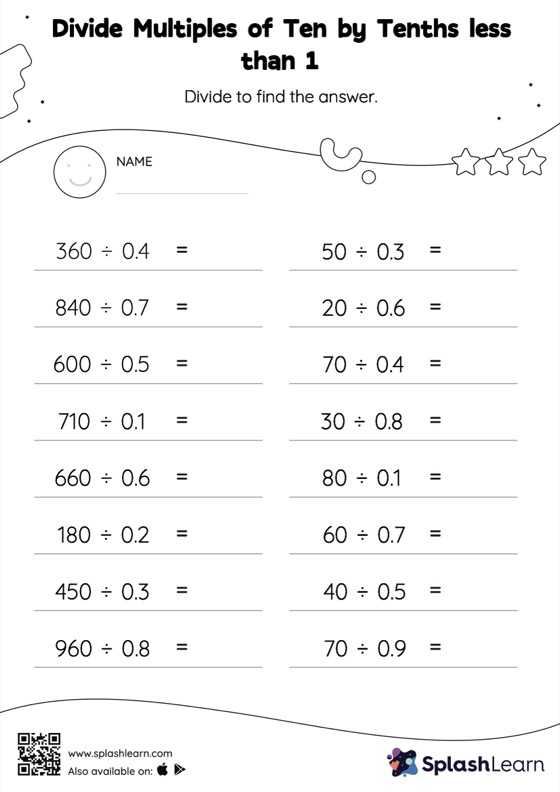# Divide Multiples of Ten by Tenths less than 1: Horizontal Division Worksheet

Home > Divide Multiples of Ten by Tenths less than 1: Horizontal DivisionThis worksheet gives students an opportunity to apply the concepts of division to divide multiples of ten by tenths less than 1. This worksheet is about practicing with the horizontal format in which numbers are written side by side. To develop flexibility with numbers and operations, students need to have enough practice in this format and not just rely on the vertical/column method.